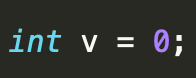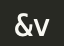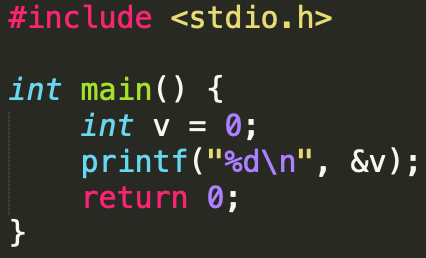# Pointers in C Programming with Examples

## Pointers in C Programming with Examples

What is a Pointer?

In simple words, a pointer is an address. It is a derived data type that stores the memory address. A pointer can also be used to refer another pointer, function. A pointer can be incremented/ decremented, i.e., to point to the next/ previous memory location.

## How does Pointer Work?

If we declare a variable v of type int, v will actually store a value.v is equal to zero now.

However, each variable, apart from value, also has its address (or, simply put, where it is located in the memory). The address can be retrieved by putting an ampersand (&) before the variable name.If you print the address of a variable on the screen, it will look like a totally random number (moreover, it can be different from run to run).

Let’s try this in practice.The output of this program is -480613588.

Now, what is a pointer? Instead of storing a value, a pointer will y store the address of a variable.

Int *y = &v;

 VARIABLE POINTER### Declaring a pointer

Like variables, pointers have to be declared before they can be used in your program. Pointers can be named anything you want as long as they obey C’s naming rules. A pointer declaration has the following form.

`data_type * pointer_variable_name;`

Here,

• data_type is the pointer’s base type of C’s variable types and indicates the type of the variable that the pointer points to.
• The asterisk (*: the same asterisk used for multiplication) which is indirection operator, declares a pointer.

Let’s see some valid pointer declarations

```int    *ptr_thing;            /* pointer to an integer */
int *ptr1,thing;/* ptr1 is a pointer to type integer and thing is an integer variable */
double    *ptr2;    /* pointer to a double */
float    *ptr3;      /* pointer to a float */
char    *ch1 ;       /* pointer to a character */
float  *ptr, variable;/*ptr is a pointer to type float and variable is an ordinary float variable */
```

### Initialize a pointer

After declaring a pointer, we initialize it like standard variables with a variable address. If pointers are not uninitialized and used in the program, the results are unpredictable and potentially disastrous.

To get the address of a variable, we use the ampersand (&)operator, placed before the name of a variable whose address we need. Pointer initialization is done with the following syntax.

` pointer = &variable; `

A simple program for pointer illustration is given below:

```#include <stdio.h>
int main()
{
int a=10;    //variable declaration
int *p;      //pointer variable declaration
p=&a;        //store address of variable a in pointer p
printf("Address stored in a variable p is:%x\n",p);  //accessing the address
printf("Value stored in a variable p is:%d\n",*p);   //accessing the value
return 0;
}
```

Output:

```Address stored in a variable p is:60ff08
Value stored in a variable p is:10
```
 Operator Meaning * Serves 2 purposeDeclaration of a pointerReturns the value of the referenced variable & Serves only 1 purposeReturns the address of a variable

## Types of a pointer

### Null pointer

We can create a null pointer by assigning null value during the pointer declaration. This method is useful when you do not have any address assigned to the pointer. A null pointer always contains value 0.

Following program illustrates the use of a null pointer:

```#include <stdio.h>
int main()
{
int *p = NULL; 	//null pointer
printf(“The value inside variable p is:\n%x”,p);
return 0;
}
```

Output:

```The value inside variable p is:
0
```

### Void Pointer

In C programming, a void pointer is also called as a generic pointer. It does not have any standard data type. A void pointer is created by using the keyword void. It can be used to store an address of any variable.

Following program illustrates the use of a void pointer:

```#include <stdio.h>
int main()
{
void *p = NULL; 	//void pointer
printf("The size of pointer is:%d\n",sizeof(p));
return 0;
}
```

Output:

```The size of pointer is:4
```

### Wild pointer

A pointer is said to be a wild pointer if it is not being initialized to anything. These types of pointers are not efficient because they may point to some unknown memory location which may cause problems in our program and it may lead to crashing of the program. One should always be careful while working with wild pointers.

Following program illustrates the use of wild pointer:

```#include <stdio.h>
int main()
{
int *p; 	//wild pointer
printf("\n%d",*p);
return 0;
}
```

Output

```timeout: the monitored command dumped core
sh: line 1: 95298 Segmentation fault      timeout 10s main
```

Other types of pointers in ‘c’ are as follows:

• Dangling pointer
• Complex pointer
• Near pointer
• Far pointer
• Huge pointer

## Direct and Indirect Access Pointers

In C, there are two equivalent ways to access and manipulate a variable content

• Direct access: we use directly the variable name
• Indirect access: we use a pointer to the variable

Let’s understand this with the help of program below

```#include <stdio.h>
/* Declare and initialize an int variable */
int var = 1;
/* Declare a pointer to int */
int *ptr;
int main( void )
{
/* Initialize ptr to point to var */
ptr = &var;
/* Access var directly and indirectly */
printf("\nDirect access, var = %d", var);
printf("\nIndirect access, var = %d", *ptr);
/* Display the address of var two ways */
printf("\n\nThe address of var = %d", &var);
printf("\nThe address of var = %d\n", ptr);
/*change the content of var through the pointer*/
*ptr=48;
printf("\nIndirect access, var = %d", *ptr);
return 0;}```

After compiling the program without any errors, the result is:

```Direct access, var = 1
Indirect access, var = 1

The address of var = 4202496
The address of var = 4202496

Indirect access, var = 48
```

## Pointers Arithmetic

The pointer operations are summarized in the following figure

### Priority operation (precedence)

When working with pointers, we must observe the following priority rules:

• The operators * and & have the same priority as the unary operators (the negation!, the incrementation++, decrement–).
• In the same expression, the unary operators *, &,!, ++, – are evaluated from right to left.

If a P pointer points to an X variable, then * P can be used wherever X can be written.

The following expressions are equivalent:

 int X =10int *P = &Y;For the above code, below expressions are true Expression Equivalent Expression Y=*P+1*P=*P+10*P+=2++*P(*P)++ Y=X+1X=X+10X+=2++XX++

In the latter case, parentheses are needed: as the unary operators * and ++ are evaluated from right to left, without the parentheses the pointer P would be incremented, not the object on which P points.

Below table shows the arithmetic and basic operation that can be used when dealing with pointers

 Operation Explanation Assignment int *P1,*P2 P1=P2; P1 and P2 point to the same integer variable Incrementation and decrementation Int *P1; P1++;P1– ; Adding an offset (Constant) This allows the pointer to move N elements in a table. The pointer will be increased or decreased by N times the number of byte (s) of the type of the variable. P1+5;

## Pointers and Arrays

Traditionally, we access the array elements using its index, but this method can be eliminated by using pointers. Pointers make it easy to access each array element.

```#include <stdio.h>
int main()
{
int a={1,2,3,4,5};   //array initialization
int *p;     //pointer declaration
/*the ptr points to the first element of the array*/

p=a; /*We can also type simply ptr==&a */

printf("Printing the array elements using pointer\n");
for(int i=0;i<5;i++)    //loop for traversing array elements
{
printf("\n%x",*p);  //printing array elements
p++;    //incrementing to the next element, you can also write p=p+1
}
return 0;
}
```

Output

```1
2
3
4
5
```

Adding a particular number to a pointer will move the pointer location to the value obtained by an addition operation. Suppose p is a pointer that currently points to the memory location 0 if we perform following addition operation, p+1 then it will execute in this manner:

Since p currently points to the location 0 after adding 1, the value will become 1, and hence the pointer will point to the memory location 1.

## Pointers and Strings

A string is an array of char objects, ending with a null character ‘\ 0’. We can manipulate strings using pointers. Here is an example that explains this section

```#include <stdio.h>
#include <string.h>
int main()
{
char str[]="Hello Guru99!";
char *p;
p=str;
printf("First character is:%c\n",*p);
p =p+1;
printf("Next character is:%c\n",*p);
printf("Printing all the characters in a string\n");
p=str;  //reset the pointer
for(int i=0;i<strlen(str);i++)
{
printf("%c\n",*p);
p++;
}
return 0;
}
```

Output

```First character is:H
Next character is:e
Printing all the characters in a string
H
e
l
l
o

G
u
r
u
9
9
!
```

Another way to deal strings is with an array of pointers like in the following program:

```#include <stdio.h>
int main(){
char *materials[ ] = {  "iron",  "copper",  "gold"};
printf("Please remember these materials :\n");
int i ;
for (i = 0; i < 3; i++) {
printf("%s\n", materials[ i ]);}
return 0;}
```

Output:

```Please remember these materials:
iron
copper
gold
```

## Advantages of Pointers

• Pointers are useful for accessing memory locations.
• Pointers provide an efficient way for accessing the elements of an array structure.
• Pointers are used for dynamic memory allocation as well as deallocation.
• Pointers are used to form complex data structures such as linked list, graph, tree, etc.

## Disadvantages of Pointers

• Pointers are a little complex to understand.
• Pointers can lead to various errors such as segmentation faults or can access a memory location which is not required at all.
• If an incorrect value is provided to a pointer, it may cause memory corruption.
• Pointers are also responsible for memory leakage.
• Pointers are comparatively slower than that of the variables.
• Programmers find it very difficult to work with the pointers; therefore it is programmer’s responsibility to manipulate a pointer carefully.

Summary

• A pointer is nothing but a memory location where data is stored.
• A pointer is used to access the memory location.
• There are various types of pointers such as a null pointer, wild pointer, void pointer and other types of pointers.
• Pointers can be used with array and string to access elements more efficiently.
• We can create function pointers to invoke a function dynamically.
• Arithmetic operations can be done on a pointer which is known as pointer arithmetic.
• Pointers can also point to function which make it easy to call different functions in the case of defining an array of pointers.
• When you want to deal different variable data type, you can use a typecast void pointer.